Courses

# Short & Long Answer Question: Chemical Bonding and Molecular- 2 Notes | EduRev

## JEE : Short & Long Answer Question: Chemical Bonding and Molecular- 2 Notes | EduRev

The document Short & Long Answer Question: Chemical Bonding and Molecular- 2 Notes | EduRev is a part of the JEE Course Chemistry for JEE.
All you need of JEE at this link: JEE

Q.51 In the following molecule: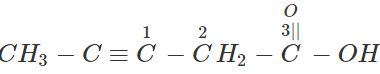arrange the carbon atoms numberedC−1,C−2  andC−3 in the decreasing order of s-character.
Hybridisation:C−1=sp,C−2=sp3,C−3=sp2 . Hence, s-character will be in the order: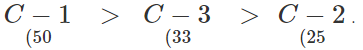Q.52 What is the hybrid state of BeCl2? What will be the change in the hybrid state of BeCl2 in the solid state?

Answer: In the vapour state at high temperature, BeCl2 exists as linear molecule, Cl−Be−Cl. The hybridization of the central atom is sp. In the solid state, it has a polymeric structure with chlorine bridges as follows: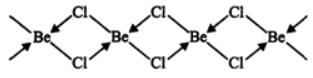Two Cl atoms are linked to Be atom by two coordinate bonds and two by covalent bonds. For these bonds to be formed. Be in the excited state with the configuration 1s22s12p1x2p0y2p0undergoes sphybridisation. Two half-filled hybrid orbitals will form normal covalent bonds with two Cl atom. The other two Cl atoms are coordinated to Be atom by donating electron pairs into the empty hybrid orbitals.
Q.53 Arrange the following in order of decreasing bond angles (i)CH4,NH3,H2O,BF3,C2H2 (ii) NH3,NH2,NH+4

Answer: (i)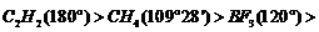NH3(107)>H2O>(104.5). (ii)NH+4>NH3>NH2 This is because all of them involve sp3 hybridization. The number of lone pair of electrons present on N-atom are 0, 1 and 2 respectively. Greater the number of lone pairs, greater are the repulsions on the bond pairs and hence smaller is the angle.
Q.54 Write the structure of an anion which is isostructural with BF3 and the structure of a cation which is isostructural with CH4

Answer: (i) NO3 (Triangular planar) (ii) NH+4 (tetrahedral)
Q.55 In SF4 molecule, the lone pair of electrons occupies an equatorial position rather than axial position in the overall trigonal bipyramidal arrangement. Why?

Answer: The lp−bp repulsions are less if it occupies equatorial position than if it occupies axial position. As a result, energy is less and stability is more
Q.56 Why axial bonds of PCl5 are longer than equatorial bonds?

Answer: This is due to greater repulsion on the axial bond pairs by the equatorial bond pairs of electrons.
Q.57 Which of the following species have same shape/same bond order? N−3,NO−2,CO2,O3

Answer: Isoelectronic species have same shape/same bond order. The number of outer electrons is same in CO2 and N−3 and same in O3 and NO−2.
Q.58 Write two resonating structures of N2O that satisfy the octet rule.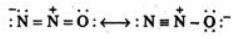Q.59 Taking  Z-axis as the inter nuclear axis, explain why 2px or 2py orbital does not combine with 2s orbital to form molecular orbitals?

Answer: The small ++ overlap cancels the +- overlap. Hence, there is no net overlapping.

Q.60 Explain why N2 has  greater bond dissociation energy than N+2 whereas O+2 has greater bond dissociation energy than O2.

Answer: B.O. of N2(3)>B.O. of N+2(2⋅5) but B.O. of O+2(2⋅5)>B.O. of O2(2). Greater the bond order, greater is the bond dissociation energy.
Q.61 Can we have a diatomic molecule with its ground state molecular orbitals full with electrons? Give a reason for your answer.

Answer: No. Because bond order becomes zero, e.g., in case of He2,Be2,Ne2, etc. Note that in H21sM.O.is full but σ1sis empty.

Q.62 Out of H and H2, the latter has higher first ionization energy while out of O and O2, the former has higher first ionization energy. Explain why.

Answer: In H2, first electron has to be removed from σ(1s) which has lower energy (more stable) than H-atom. In O2, first electron has to be removed from π(2px) or π(2py) orbital which has higher energy than 2 p-orbital of O-atom.
Q.63 Give reason for the following: H+2 and H2 ions have the same bond order but H+2 ions are more stable than H−2.

Answer: H−2 has one electron in the anti bonding σ1s molecular orbital due to which there is some destabilizing effect and hence is less stable than H+2 ion (which has no electron in the anti bonding orbital).
Q.64 Compare the relative stabilities of O−2 and N+2 and comment on their magnetic (paramagnetic or diamagnetic) behaviour.
M.O. Electronic configuration of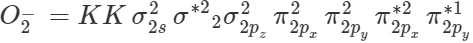Bond order=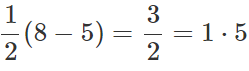M.O. Electronic configuration of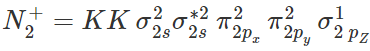Bond order =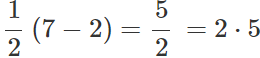As bond order of N+2> bond order of O2, therefore, N+2 is more stable than O2. Each of them contains unpaired electron, hence both are paramagnetic.
Q.65 (a) How bond energy varies from N−2 to N+and why? (b) On the basis of molecular orbital theory what is similarity between (i) F2,O−−2(ii) CO,N2,NO+?

Answer: (a) Bond energy of N+2= Bond energy of N−2. This is because they have the same bond order. (Strictly speaking, N−2 is slightly less stable and hence has less bond energy than N+2 due to presence of greater number of electrons in the anti bonding molecular orbitals) (b) (i) Same bond order and bond length, (ii) Same bond order and bond length.
Q.66 N2 has higher bond order than NO. Explain.

Answer: Bond order of N2=3 . Bond order of NO=2 1/2
Q.67 Why KHF2 exists but KHCl2 does not?

Answer: Due to H-bonding in HF, we have H−F------H−F------H−F------ This can dissociate to give HF−2 ion and hence KHF2 exists but there is no H-bonding in H−Cl. So HCl−2 ion does not exist and hence KHCl2 also does not exist.
Q.68 When we move from HF to HCl,  the boiling point drops sharply but on moving further to HBr  and HI the boiling point increases. Why? or Out of HF,HCl,HBr  and HI, which has lowest boiling point and why?

Answer: In H−F there is H-bonding. Hence, the boiling point is high. There is no H-bonding in HCl. So the boiling point is less. On moving further to HBr and HI, the size of the molecule increases and so the van der Waals forces increase and so does the boiling point. Hence, HCl has the lowest boiling point.
Q.69 Ethanol has higher boiling point than diethyl ether or ethyl amine. Why?

Answer: In ethanol, there is H-bonding but in diethyl ether, there is no H-bonding (because O-atom is attached to C-atom) and in case of ethyl amine, the H-bonds formed by N-atom are weaker than those formed by O-atom.
Q.70 How many H-bonds are formed by each H2O molecule and how many water molecules are attached to each water molecule and in what direction?

Answer: Each H2O molecule forms four H-bonds, two with O-atom and two with H-atoms. Further, each H2O molecule is linked to four H2O molecules through H-bonds tetrahedrally.
Q.71 Out of o-nitro phenol and p-nitro phenol, which has higher boiling point and why?

Answer: p-nitro phenol has higher boiling point because there is intermolecular H-bonding while in o-nitro phenol, there is intra molecular H-bonding.
Q.72 Though Cl has nearly same electro negativity as N, yet there is no H-bonding in HCl. Why?

Answer: Chlorine atom has a large size.
Q.73 Why glucose, fructose, sucrose etc. are soluble in water though they are covalent compounds?

Answer: These compounds contain polar -OH groups which can form H-bonds with water.
Q.74 Explain why HF is less viscous than H2O.

Answer: There is greater intermolecular hydrogen bonding in H2O than that in HF as each H2O molecule forms four H-bonds with other water molecules whereas HF forms only two H- bonds with other HF molecules. Greater the intermolecular H- bonding, greater is the viscosity. Hence, HF is less viscous than H2O.
Q.75 From each of the following pairs, select the molecule with higher value of the property mentioned against each pair: (i)NH3,PH3: bond angle (ii) NF3,NH3: dipole moment (iii) MgO,CaO: hardness (iv) HCl,HBr: ionic character

Answer: (i) NH3 (ii) NH3 (iii) MgO (iv) HCl
Q.76 Account for the following: The experimentally determined N−F bond length in NF3 is greater than the sum of the single covalent radii of N and F.

Answer: This is because both N and F are small and hence have high electron density. So they repel the bond pairs thereby making the N-F bond length larger.

Q.77 Why NaCl is a bad conductor of electricity in the solid state?

Answer: In the solid state, Na+and Cl ions are not free.
Q.78 Why two hydrogen atoms combine to form H2 but two helium atoms do not combine to form He2?
on the basis of (i) New forces of attraction and repulsion and (ii) orbital concept).

Offer running on EduRev: Apply code STAYHOME200 to get INR 200 off on our premium plan EduRev Infinity!

## Chemistry for JEE

204 videos|438 docs|333 tests

,

,

,

,

,

,

,

,

,

,

,

,

,

,

,

,

,

,

,

,

,

;